# 可视化分析

## 流程图

``````from IPython.display import Image, display
Image('images/13_visual_analysis_flowchart.png')
``````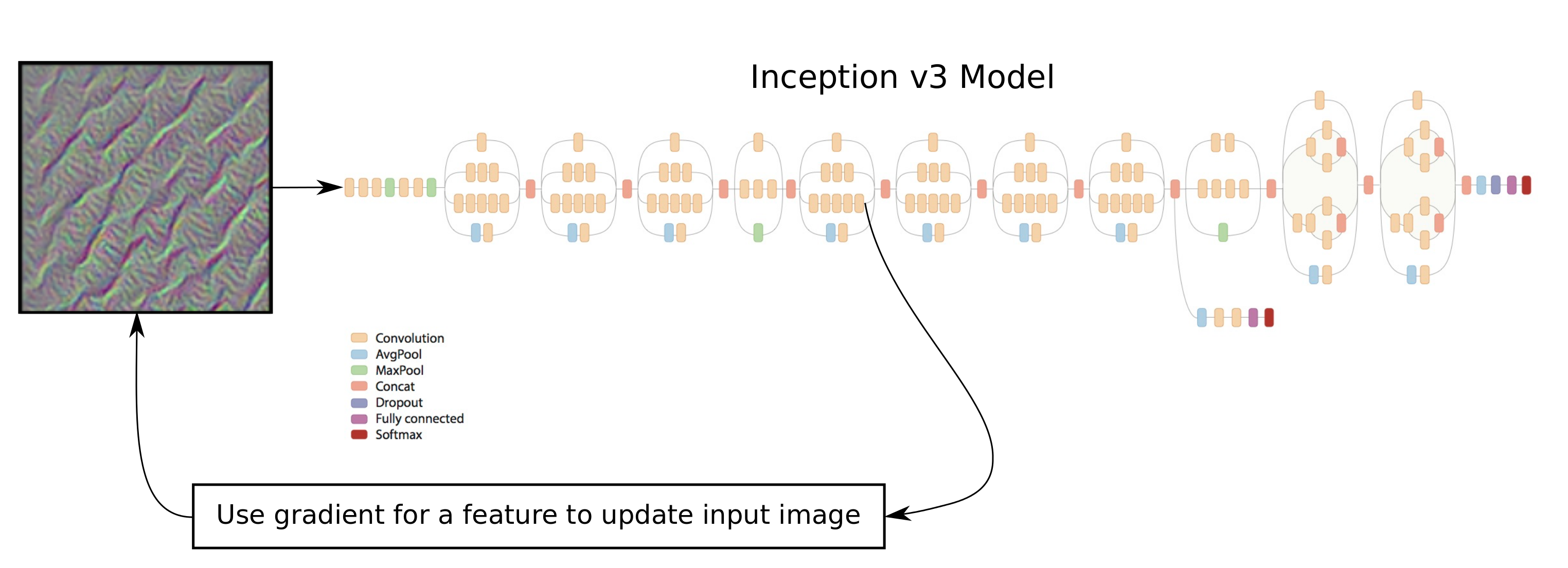## 导入

``````%matplotlib inline
import matplotlib.pyplot as plt
import tensorflow as tf
import numpy as np

import inception
``````

``````tf.__version__
``````
``````'1.1.0'
``````

## Inception 模型

### 从网上下载Inception模型

``````# inception.data_dir = 'inception/'
``````

``````inception.maybe_download()
``````
``````Downloading Inception v3 Model ...
Done.
``````

### 卷积层的名称

``````def get_conv_layer_names():
model = inception.Inception()

# Create a list of names for the operations in the graph
# for the Inception model where the operator-type is 'Conv2D'.
names = [op.name for op in model.graph.get_operations() if op.type=='Conv2D']

# Close the TensorFlow session inside the model-object.
model.close()

return names
``````
``````conv_names = get_conv_layer_names()
``````

``````len(conv_names)
``````
``````94
``````

``````conv_names[:5]
``````
``````['conv/Conv2D',
'conv_1/Conv2D',
'conv_2/Conv2D',
'conv_3/Conv2D',
'conv_4/Conv2D']
``````

``````conv_names[-5:]
``````
``````['mixed_10/tower_1/conv/Conv2D',
'mixed_10/tower_1/conv_1/Conv2D',
'mixed_10/tower_1/mixed/conv/Conv2D',
'mixed_10/tower_1/mixed/conv_1/Conv2D',
'mixed_10/tower_2/conv/Conv2D']
``````

## 找到输入图像的帮助函数

``````def optimize_image(conv_id=None, feature=0,
num_iterations=30, show_progress=True):
"""
Find an image that maximizes the feature
given by the conv_id and feature number.

Parameters:
conv_id: Integer identifying the convolutional layer to
maximize. It is an index into conv_names.
If None then use the last fully-connected layer
before the softmax output.
feature: Index into the layer for the feature to maximize.
num_iteration: Number of optimization iterations to perform.
show_progress: Boolean whether to show the progress.
"""

# Load the Inception model. This is done for each call of
# this function because we will add a lot to the graph
# which will cause the graph to grow and eventually the
# computer will run out of memory.
model = inception.Inception()

# Reference to the tensor that takes the raw input image.
resized_image = model.resized_image

# Reference to the tensor for the predicted classes.
# This is the output of the final layer's softmax classifier.
y_pred = model.y_pred

# Create the loss-function that must be maximized.
if conv_id is None:
# If we want to maximize a feature on the last layer,
# then we use the fully-connected layer prior to the
# softmax-classifier. The feature no. is the class-number
# and must be an integer between 1 and 1000.
# The loss-function is just the value of that feature.
loss = model.y_logits[0, feature]
else:
# If instead we want to maximize a feature of a
# convolutional layer inside the neural network.

# Get the name of the convolutional operator.
conv_name = conv_names[conv_id]

# Get a reference to the tensor that is output by the
# operator. Note that ":0" is added to the name for this.
tensor = model.graph.get_tensor_by_name(conv_name + ":0")

# Set the Inception model's graph as the default
# so we can add an operator to it.
with model.graph.as_default():
# The loss-function is the average of all the
# tensor-values for the given feature. This
# ensures that we generate the whole input image.
# You can try and modify this so it only uses
# a part of the tensor.
loss = tf.reduce_mean(tensor[:,:,:,feature])

# Get the gradient for the loss-function with regard to
# the resized input image. This creates a mathematical
# function for calculating the gradient.

# Create a TensorFlow session so we can run the graph.
session = tf.Session(graph=model.graph)

# Generate a random image of the same size as the raw input.
# Each pixel is a small random value between 128 and 129,
# which is about the middle of the colour-range.
image_shape = resized_image.get_shape()
image = np.random.uniform(size=image_shape) + 128.0

# Perform a number of optimization iterations to find
# the image that maximizes the loss-function.
for i in range(num_iterations):
# Create a feed-dict. This feeds the image to the
# tensor in the graph that holds the resized image, because
# this is the final stage for inputting raw image data.
feed_dict = {model.tensor_name_resized_image: image}

# Calculate the predicted class-scores,
# as well as the gradient and the loss-value.
feed_dict=feed_dict)

# Squeeze the dimensionality for the gradient-array.

# The gradient now tells us how much we need to change the
# input image in order to maximize the given feature.

# Calculate the step-size for updating the image.
# This step-size was found to give fast convergence.
# The addition of 1e-8 is to protect from div-by-zero.
step_size = 1.0 / (grad.std() + 1e-8)

# This is called gradient ascent.

# Ensure all pixel-values in the image are between 0 and 255.
image = np.clip(image, 0.0, 255.0)

if show_progress:
print("Iteration:", i)

# Convert the predicted class-scores to a one-dim array.
pred = np.squeeze(pred)

# The predicted class for the Inception model.
pred_cls = np.argmax(pred)

# Name of the predicted class.
cls_name = model.name_lookup.cls_to_name(pred_cls,
only_first_name=True)

# The score (probability) for the predicted class.
cls_score = pred[pred_cls]

# Print the predicted score etc.
msg = "Predicted class-name: {0} (#{1}), score: {2:>7.2%}"
print(msg.format(cls_name, pred_cls, cls_score))

# Print statistics for the gradient.
msg = "Gradient min: {0:>9.6f}, max: {1:>9.6f}, stepsize: {2:>9.2f}"

# Print the loss-value.
print("Loss:", loss_value)

# Newline.
print()

# Close the TensorFlow session inside the model-object.
model.close()

return image.squeeze()
``````

### 绘制图像和噪声的帮助函数

``````def normalize_image(x):
# Get the min and max values for all pixels in the input.
x_min = x.min()
x_max = x.max()

# Normalize so all values are between 0.0 and 1.0
x_norm = (x - x_min) / (x_max - x_min)

return x_norm
``````

``````def plot_image(image):
# Normalize the image so pixels are between 0.0 and 1.0
img_norm = normalize_image(image)

# Plot the image.
plt.imshow(img_norm, interpolation='nearest')
plt.show()
``````

``````def plot_images(images, show_size=100):
"""
The show_size is the number of pixels to show for each image.
The max value is 299.
"""

# Create figure with sub-plots.
fig, axes = plt.subplots(2, 3)

# Use interpolation to smooth pixels?
smooth = True

# Interpolation type.
if smooth:
interpolation = 'spline16'
else:
interpolation = 'nearest'

# For each entry in the grid.
for i, ax in enumerate(axes.flat):
# Get the i'th image and only use the desired pixels.
img = images[i, 0:show_size, 0:show_size, :]

# Normalize the image so its pixels are between 0.0 and 1.0
img_norm = normalize_image(img)

# Plot the image.
ax.imshow(img_norm, interpolation=interpolation)

# Remove ticks.
ax.set_xticks([])
ax.set_yticks([])

# Ensure the plot is shown correctly with multiple plots
# in a single Notebook cell.
plt.show()
``````

### 优化和绘制图像的帮助函数

``````def optimize_images(conv_id=None, num_iterations=30, show_size=100):
"""
Find 6 images that maximize the 6 first features in the layer
given by the conv_id.

Parameters:
conv_id: Integer identifying the convolutional layer to
maximize. It is an index into conv_names.
If None then use the last layer before the softmax output.
num_iterations: Number of optimization iterations to perform.
show_size: Number of pixels to show for each image. Max 299.
"""

# Which layer are we using?
if conv_id is None:
print("Final fully-connected layer before softmax.")
else:
print("Layer:", conv_names[conv_id])

# Initialize the array of images.
images = []

# For each feature do the following. Note that the
# last fully-connected layer only supports numbers
# between 1 and 1000, while the convolutional layers
# support numbers between 0 and some other number.
# So we just use the numbers between 1 and 7.
for feature in range(1,7):
print("Optimizing image for feature no.", feature)

# Find the image that maximizes the given feature
# for the network layer identified by conv_id (or None).
image = optimize_image(conv_id=conv_id, feature=feature,
show_progress=False,
num_iterations=num_iterations)

# Squeeze the dim of the array.
image = image.squeeze()

# Append to the list of images.
images.append(image)

# Convert to numpy-array so we can index all dimensions easily.
images = np.array(images)

# Plot the images.
plot_images(images=images, show_size=show_size)
``````

## 结果

### 为浅处的卷积层优化图像

``````image = optimize_image(conv_id=5, feature=2,
num_iterations=30, show_progress=True)
``````
``````Iteration: 0
Predicted class-name: dishwasher (#667), score:   4.81%
Gradient min: -0.000083, max:  0.000100, stepsize:  76290.32
Loss: 4.83793

Iteration: 1
Predicted class-name: kite (#397), score:  15.12%
Gradient min: -0.000142, max:  0.000126, stepsize:  71463.42
Loss: 5.59611

Iteration: 2
Predicted class-name: wall clock (#524), score:   6.85%
Gradient min: -0.000119, max:  0.000121, stepsize:  80427.39
Loss: 6.91725

Iteration: 3
Predicted class-name: syringe (#531), score:   4.69%
Gradient min: -0.000124, max:  0.000116, stepsize:  87046.41
Loss: 7.93267

Iteration: 4
Predicted class-name: syringe (#531), score:   6.53%
Gradient min: -0.000115, max:  0.000122, stepsize:  94634.06
Loss: 8.85594

Iteration: 5
Predicted class-name: syringe (#531), score:  21.31%
Gradient min: -0.000108, max:  0.000131, stepsize: 103182.49
Loss: 9.70698

Iteration: 6
Predicted class-name: syringe (#531), score:  36.39%
Gradient min: -0.000102, max:  0.000099, stepsize: 111440.73
Loss: 10.4558

Iteration: 7
Predicted class-name: syringe (#531), score:  43.79%
Gradient min: -0.000100, max:  0.000083, stepsize: 119285.09
Loss: 11.1371

Iteration: 8
Predicted class-name: syringe (#531), score:  34.85%
Gradient min: -0.000078, max:  0.000098, stepsize: 126258.06
Loss: 11.7331

Iteration: 9
Predicted class-name: syringe (#531), score:  18.28%
Gradient min: -0.000075, max:  0.000071, stepsize: 133766.53
Loss: 12.2777

Iteration: 10
Predicted class-name: syringe (#531), score:  11.91%
Gradient min: -0.000072, max:  0.000079, stepsize: 139181.44
Loss: 12.7673

Iteration: 11
Predicted class-name: binder (#835), score:  13.27%
Gradient min: -0.000079, max:  0.000070, stepsize: 145263.47
Loss: 13.2062

Iteration: 12
Predicted class-name: binder (#835), score:  15.05%
Gradient min: -0.000060, max:  0.000101, stepsize: 150589.72
Loss: 13.6149

Iteration: 13
Predicted class-name: binder (#835), score:  14.79%
Gradient min: -0.000074, max:  0.000072, stepsize: 156626.62
Loss: 13.9922

Iteration: 14
Predicted class-name: binder (#835), score:  14.44%
Gradient min: -0.000078, max:  0.000062, stepsize: 160979.04
Loss: 14.3428

Iteration: 15
Predicted class-name: binder (#835), score:  11.76%
Gradient min: -0.000081, max:  0.000081, stepsize: 164249.60
Loss: 14.6689

Iteration: 16
Predicted class-name: binder (#835), score:   9.61%
Gradient min: -0.000069, max:  0.000073, stepsize: 169375.77
Loss: 14.968

Iteration: 17
Predicted class-name: binder (#835), score:   7.51%
Gradient min: -0.000060, max:  0.000086, stepsize: 173951.43
Loss: 15.2644

Iteration: 18
Predicted class-name: binder (#835), score:   6.16%
Gradient min: -0.000057, max:  0.000074, stepsize: 176921.49
Loss: 15.5303

Iteration: 19
Predicted class-name: quilt (#976), score:   6.22%
Gradient min: -0.000067, max:  0.000068, stepsize: 182788.52
Loss: 15.7967

Iteration: 20
Predicted class-name: quilt (#976), score:   7.31%
Gradient min: -0.000068, max:  0.000063, stepsize: 185266.16
Loss: 16.0442

Iteration: 21
Predicted class-name: bib (#941), score:   7.74%
Gradient min: -0.000048, max:  0.000066, stepsize: 190195.76
Loss: 16.2883

Iteration: 22
Predicted class-name: bib (#941), score:   9.43%
Gradient min: -0.000060, max:  0.000047, stepsize: 192709.62
Loss: 16.5165

Iteration: 23
Predicted class-name: bib (#941), score:  11.05%
Gradient min: -0.000064, max:  0.000049, stepsize: 197288.09
Loss: 16.7361

Iteration: 24
Predicted class-name: bib (#941), score:  12.59%
Gradient min: -0.000054, max:  0.000047, stepsize: 201010.69
Loss: 16.9544

Iteration: 25
Predicted class-name: bib (#941), score:  15.13%
Gradient min: -0.000045, max:  0.000049, stepsize: 204798.67
Loss: 17.1659

Iteration: 26
Predicted class-name: bib (#941), score:  15.91%
Gradient min: -0.000047, max:  0.000047, stepsize: 208499.70
Loss: 17.3637

Iteration: 27
Predicted class-name: bib (#941), score:  17.96%
Gradient min: -0.000056, max:  0.000059, stepsize: 210286.13
Loss: 17.559

Iteration: 28
Predicted class-name: bib (#941), score:  19.26%
Gradient min: -0.000043, max:  0.000043, stepsize: 214742.82
Loss: 17.7469

Iteration: 29
Predicted class-name: bib (#941), score:  18.87%
Gradient min: -0.000047, max:  0.000059, stepsize: 218511.00
Loss: 17.9321
``````
``````plot_image(image)
``````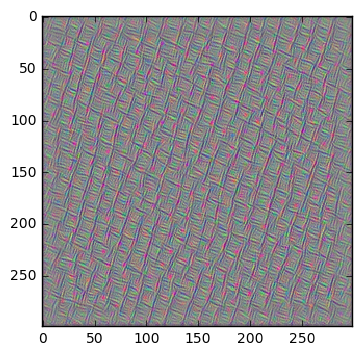### 为卷积层优化多张图像

``````optimize_images(conv_id=0, num_iterations=10)
``````
``````Layer: conv/Conv2D
Optimizing image for feature no. 1
Optimizing image for feature no. 2
Optimizing image for feature no. 3
Optimizing image for feature no. 4
Optimizing image for feature no. 5
``````
``````optimize_images(conv_id=3, num_iterations=30)
``````
``````Layer: conv_3/Conv2D
Optimizing image for feature no. 1
Optimizing image for feature no. 2
Optimizing image for feature no. 3
Optimizing image for feature no. 4
Optimizing image for feature no. 5
Optimizing image for feature no. 6
``````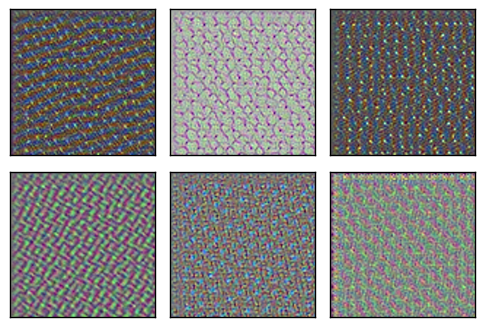``````optimize_images(conv_id=4, num_iterations=30)
``````
``````Layer: conv_4/Conv2D
Optimizing image for feature no. 1
Optimizing image for feature no. 2
Optimizing image for feature no. 3
Optimizing image for feature no. 4
Optimizing image for feature no. 5
Optimizing image for feature no. 6
````````````optimize_images(conv_id=5, num_iterations=30)
``````
``````Layer: mixed/conv/Conv2D
Optimizing image for feature no. 1
Optimizing image for feature no. 2
Optimizing image for feature no. 3
Optimizing image for feature no. 4
Optimizing image for feature no. 5
Optimizing image for feature no. 6
``````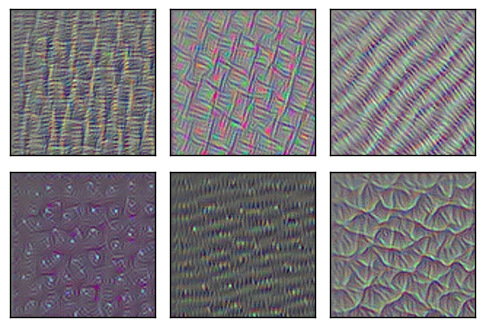``````optimize_images(conv_id=6, num_iterations=30)
``````
``````Layer: mixed/tower/conv/Conv2D
Optimizing image for feature no. 1
Optimizing image for feature no. 2
Optimizing image for feature no. 3
Optimizing image for feature no. 4
Optimizing image for feature no. 5
Optimizing image for feature no. 6
``````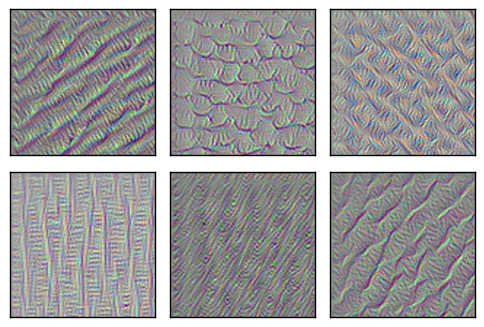``````optimize_images(conv_id=7, num_iterations=30)
``````
``````Layer: mixed/tower/conv_1/Conv2D
Optimizing image for feature no. 1
Optimizing image for feature no. 2
Optimizing image for feature no. 3
Optimizing image for feature no. 4
Optimizing image for feature no. 5
Optimizing image for feature no. 6
``````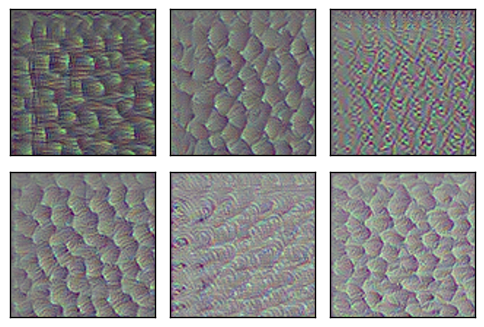``````optimize_images(conv_id=8, num_iterations=30)
``````
``````Layer: mixed/tower_1/conv/Conv2D
Optimizing image for feature no. 1
Optimizing image for feature no. 2
Optimizing image for feature no. 3
Optimizing image for feature no. 4
Optimizing image for feature no. 5
Optimizing image for feature no. 6
``````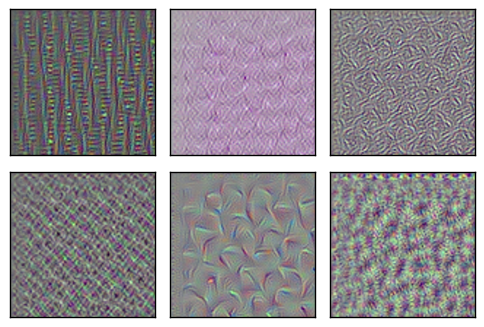``````optimize_images(conv_id=9, num_iterations=30)
``````
``````Layer: mixed/tower_1/conv_1/Conv2D
Optimizing image for feature no. 1
Optimizing image for feature no. 2
Optimizing image for feature no. 3
Optimizing image for feature no. 4
Optimizing image for feature no. 5
Optimizing image for feature no. 6
``````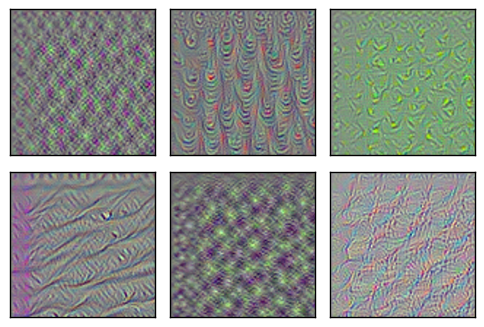``````optimize_images(conv_id=10, num_iterations=30)
``````
``````Layer: mixed/tower_1/conv_2/Conv2D
Optimizing image for feature no. 1
Optimizing image for feature no. 2
Optimizing image for feature no. 3
Optimizing image for feature no. 4
Optimizing image for feature no. 5
Optimizing image for feature no. 6
``````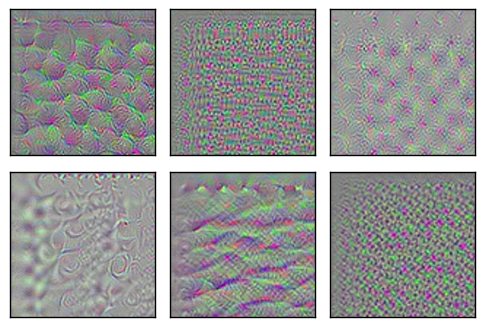``````optimize_images(conv_id=20, num_iterations=30)
``````
``````Layer: mixed_2/tower/conv/Conv2D
Optimizing image for feature no. 1
Optimizing image for feature no. 2
Optimizing image for feature no. 3
Optimizing image for feature no. 4
Optimizing image for feature no. 5
Optimizing image for feature no. 6
``````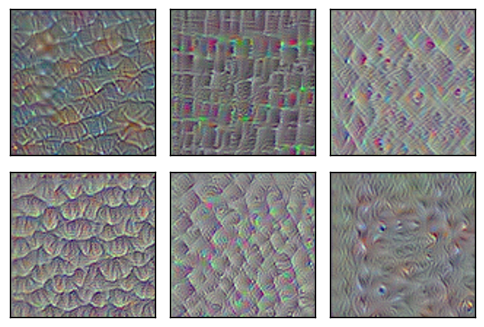``````optimize_images(conv_id=30, num_iterations=30)
``````
``````Layer: mixed_4/conv/Conv2D
Optimizing image for feature no. 1
Optimizing image for feature no. 2
Optimizing image for feature no. 3
Optimizing image for feature no. 4
Optimizing image for feature no. 5
Optimizing image for feature no. 6
``````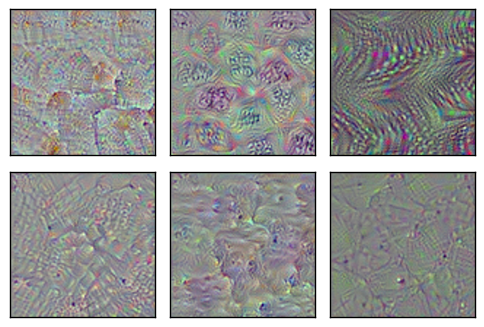``````optimize_images(conv_id=40, num_iterations=30)
``````
``````Layer: mixed_5/conv/Conv2D
Optimizing image for feature no. 1
Optimizing image for feature no. 2
Optimizing image for feature no. 3
Optimizing image for feature no. 4
Optimizing image for feature no. 5
Optimizing image for feature no. 6
``````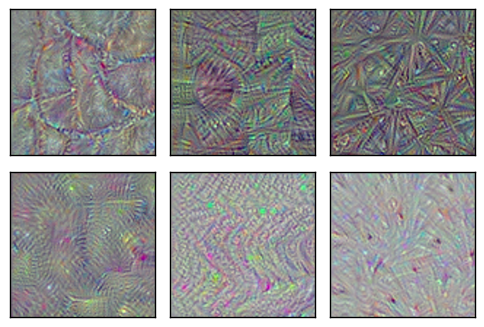``````optimize_images(conv_id=50, num_iterations=30)
``````
``````Layer: mixed_6/conv/Conv2D
Optimizing image for feature no. 1
Optimizing image for feature no. 2
Optimizing image for feature no. 3
Optimizing image for feature no. 4
Optimizing image for feature no. 5
Optimizing image for feature no. 6
``````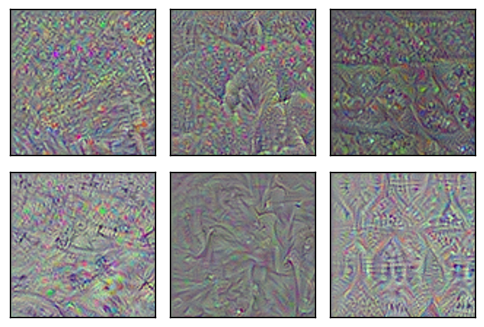``````optimize_images(conv_id=60, num_iterations=30)
``````
``````Layer: mixed_7/conv/Conv2D
Optimizing image for feature no. 1
Optimizing image for feature no. 2
Optimizing image for feature no. 3
Optimizing image for feature no. 4
Optimizing image for feature no. 5
Optimizing image for feature no. 6
``````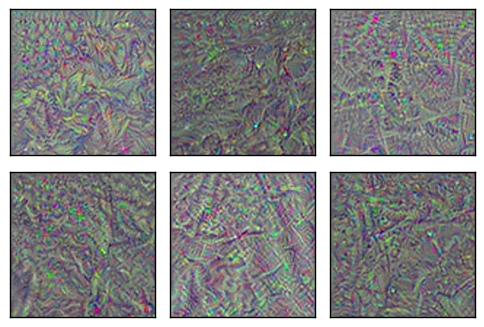``````optimize_images(conv_id=70, num_iterations=30)
``````
``````Layer: mixed_8/tower/conv/Conv2D
Optimizing image for feature no. 1
Optimizing image for feature no. 2
Optimizing image for feature no. 3
Optimizing image for feature no. 4
Optimizing image for feature no. 5
Optimizing image for feature no. 6
``````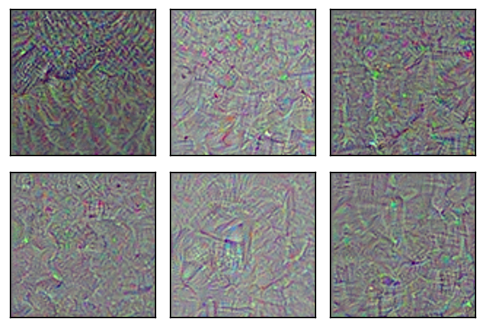``````optimize_images(conv_id=80, num_iterations=30)
``````
``````Layer: mixed_9/tower_1/conv/Conv2D
Optimizing image for feature no. 1
Optimizing image for feature no. 2
Optimizing image for feature no. 3
Optimizing image for feature no. 4
Optimizing image for feature no. 5
Optimizing image for feature no. 6
``````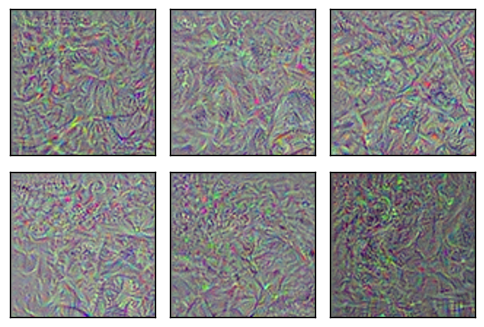``````optimize_images(conv_id=90, num_iterations=30)
``````
``````Layer: mixed_10/tower_1/conv_1/Conv2D
Optimizing image for feature no. 1
Optimizing image for feature no. 2
Optimizing image for feature no. 3
Optimizing image for feature no. 4
Optimizing image for feature no. 5
Optimizing image for feature no. 6
``````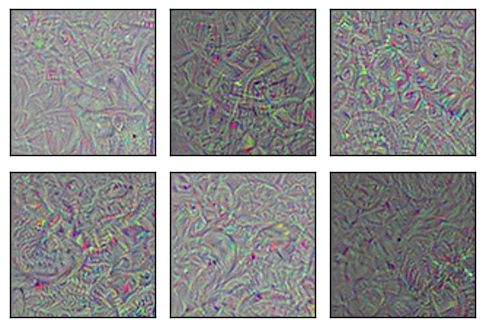``````optimize_images(conv_id=93, num_iterations=30)
``````
``````Layer: mixed_10/tower_2/conv/Conv2D
Optimizing image for feature no. 1
Optimizing image for feature no. 2
Optimizing image for feature no. 3
Optimizing image for feature no. 4
Optimizing image for feature no. 5
Optimizing image for feature no. 6
``````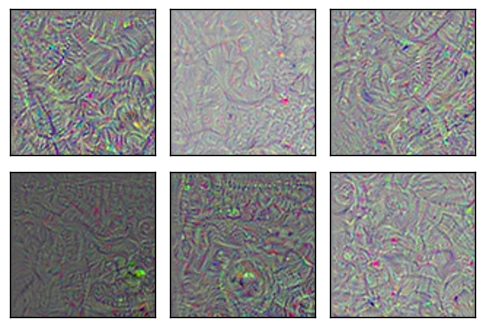### Softmax前最终的全连接层

``````optimize_images(conv_id=None, num_iterations=30)
``````
``````Final fully-connected layer before softmax.
Optimizing image for feature no. 1
Optimizing image for feature no. 2
Optimizing image for feature no. 3
Optimizing image for feature no. 4
Optimizing image for feature no. 5
Optimizing image for feature no. 6
``````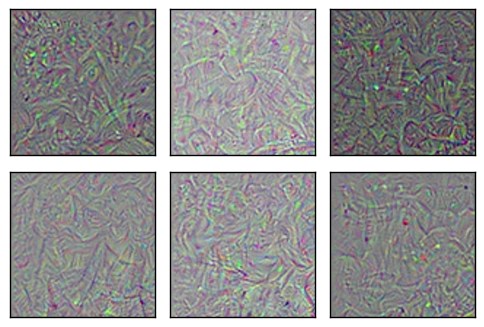Inception模型以大约100%的确信度将结果图像分类成“敏狐”，但在人眼看来，图像只是一些抽象的图案。

``````image = optimize_image(conv_id=None, feature=1,
num_iterations=100, show_progress=True)
``````
``````Iteration: 0
Predicted class-name: dishwasher (#667), score:   4.98%
Gradient min: -0.006252, max:  0.004451, stepsize:   3734.48
Loss: -0.837608

Iteration: 1
Predicted class-name: ballpoint (#907), score:   8.52%
Gradient min: -0.007303, max:  0.006427, stepsize:   2152.89
Loss: -0.416723

Iteration: 2
Predicted class-name: spider web (#600), score:  90.44%
Gradient min: -0.007480, max:  0.012272, stepsize:   1343.66
Loss: 2.77814

Iteration: 3
Predicted class-name: pot (#838), score:   3.01%
Gradient min: -0.009853, max:  0.007638, stepsize:   1526.98
Loss: 3.27751

Iteration: 4
Predicted class-name: American egret (#426), score:  11.55%
Gradient min: -0.008507, max:  0.006308, stepsize:   1787.96
Loss: 5.95497

Iteration: 5
Predicted class-name: spider web (#600), score:  21.98%
Gradient min: -0.010415, max:  0.009410, stepsize:   1722.27
Loss: 5.07394

Iteration: 6
Predicted class-name: kit fox (#1), score:  29.21%
Gradient min: -0.009298, max:  0.007885, stepsize:   2471.91
Loss: 7.98241

Iteration: 7
Predicted class-name: brain coral (#649), score:   8.95%
Gradient min: -0.004683, max:  0.004366, stepsize:   2876.78
Loss: 2.61856

Iteration: 8
Predicted class-name: kit fox (#1), score:  30.05%
Gradient min: -0.008918, max:  0.006374, stepsize:   2243.47
Loss: 8.18703

Iteration: 9
Predicted class-name: kit fox (#1), score:  55.10%
Gradient min: -0.041977, max:  0.025564, stepsize:   1270.96
Loss: 9.4695

Iteration: 10
Predicted class-name: kit fox (#1), score:  45.79%
Gradient min: -0.025474, max:  0.026726, stepsize:    858.88
Loss: 8.49094

Iteration: 11
Predicted class-name: kit fox (#1), score:  71.39%
Gradient min: -0.021939, max:  0.016643, stepsize:   1316.54
Loss: 12.4999

Iteration: 12
Predicted class-name: kit fox (#1), score:  82.78%
Gradient min: -0.011797, max:  0.017714, stepsize:   1763.08
Loss: 11.1421

Iteration: 13
Predicted class-name: kit fox (#1), score:  67.38%
Gradient min: -0.016686, max:  0.015832, stepsize:   1908.94
Loss: 11.186

Iteration: 14
Predicted class-name: kit fox (#1), score:  57.64%
Gradient min: -0.017312, max:  0.014563, stepsize:   1412.06
Loss: 9.67373

Iteration: 15
Predicted class-name: kit fox (#1), score:  69.09%
Gradient min: -0.005773, max:  0.005870, stepsize:   3163.64
Loss: 12.9428

Iteration: 16
Predicted class-name: kit fox (#1), score:  82.98%
Gradient min: -0.020765, max:  0.017950, stepsize:   1225.38
Loss: 10.6559

Iteration: 17
Predicted class-name: kit fox (#1), score:  99.04%
Gradient min: -0.005492, max:  0.006126, stepsize:   2520.72
Loss: 16.579

Iteration: 18
Predicted class-name: kit fox (#1), score:  86.78%
Gradient min: -0.017253, max:  0.028574, stepsize:   1280.11
Loss: 11.7084

Iteration: 19
Predicted class-name: kit fox (#1), score:  96.57%
Gradient min: -0.007056, max:  0.006660, stepsize:   1838.04
Loss: 17.8698

Iteration: 20
Predicted class-name: kit fox (#1), score:  99.04%
Gradient min: -0.008916, max:  0.008408, stepsize:   2720.73
Loss: 17.922

Iteration: 21
Predicted class-name: kit fox (#1), score:  68.39%
Gradient min: -0.012104, max:  0.013627, stepsize:   1398.73
Loss: 12.5718

Iteration: 22
Predicted class-name: kit fox (#1), score:  98.38%
Gradient min: -0.007660, max:  0.007840, stepsize:   2043.20
Loss: 14.0164

Iteration: 23
Predicted class-name: kit fox (#1), score:  98.36%
Gradient min: -0.009233, max:  0.006748, stepsize:   1951.74
Loss: 19.118

Iteration: 24
Predicted class-name: kit fox (#1), score:  99.44%
Gradient min: -0.013526, max:  0.015166, stepsize:   1557.67
Loss: 23.2171

Iteration: 25
Predicted class-name: kit fox (#1), score:  99.83%
Gradient min: -0.005306, max:  0.006063, stepsize:   2142.04
Loss: 21.0666

Iteration: 26
Predicted class-name: kit fox (#1), score:  99.85%
Gradient min: -0.005931, max:  0.005094, stepsize:   2287.80
Loss: 21.2772

Iteration: 27
Predicted class-name: kit fox (#1), score:  97.91%
Gradient min: -0.008425, max:  0.010999, stepsize:   1633.57
Loss: 23.1276

Iteration: 28
Predicted class-name: kit fox (#1), score:  99.98%
Gradient min: -0.012720, max:  0.010505, stepsize:   1749.55
Loss: 25.9384

Iteration: 29
Predicted class-name: kit fox (#1), score:  99.90%
Gradient min: -0.020819, max:  0.023275, stepsize:   1026.48
Loss: 22.4687

Iteration: 30
Predicted class-name: kit fox (#1), score:  99.87%
Gradient min: -0.005569, max:  0.007158, stepsize:   2436.42
Loss: 21.3727

Iteration: 31
Predicted class-name: kit fox (#1), score:  97.25%
Gradient min: -0.010902, max:  0.007087, stepsize:   1689.47
Loss: 13.2659

Iteration: 32
Predicted class-name: kit fox (#1), score:  99.96%
Gradient min: -0.006695, max:  0.006514, stepsize:   2277.89
Loss: 23.113

Iteration: 33
Predicted class-name: kit fox (#1), score:  99.89%
Gradient min: -0.011343, max:  0.011963, stepsize:   1713.67
Loss: 20.4645

Iteration: 34
Predicted class-name: kit fox (#1), score:  99.83%
Gradient min: -0.005129, max:  0.005226, stepsize:   2531.23
Loss: 22.9016

Iteration: 35
Predicted class-name: kit fox (#1), score:  99.96%
Gradient min: -0.004618, max:  0.005916, stepsize:   1979.85
Loss: 19.6406

Iteration: 36
Predicted class-name: kit fox (#1), score:  99.94%
Gradient min: -0.005298, max:  0.007882, stepsize:   2158.99
Loss: 26.6898

Iteration: 37
Predicted class-name: kit fox (#1), score:  99.77%
Gradient min: -0.009913, max:  0.010110, stepsize:   1643.65
Loss: 21.2908

Iteration: 38
Predicted class-name: kit fox (#1), score:  99.99%
Gradient min: -0.005472, max:  0.004434, stepsize:   2654.99
Loss: 28.4096

Iteration: 39
Predicted class-name: kit fox (#1), score:  99.99%
Gradient min: -0.006044, max:  0.007171, stepsize:   1646.69
Loss: 32.005

Iteration: 40
Predicted class-name: kit fox (#1), score: 100.00%
Gradient min: -0.007782, max:  0.007306, stepsize:   1853.00
Loss: 34.7635

Iteration: 41
Predicted class-name: kit fox (#1), score:  99.94%
Gradient min: -0.030789, max:  0.017443, stepsize:   1224.15
Loss: 32.2997

Iteration: 42
Predicted class-name: kit fox (#1), score: 100.00%
Gradient min: -0.005752, max:  0.006784, stepsize:   2148.87
Loss: 34.8329

Iteration: 43
Predicted class-name: kit fox (#1), score:  99.98%
Gradient min: -0.005747, max:  0.005908, stepsize:   2058.32
Loss: 33.3857

Iteration: 44
Predicted class-name: kit fox (#1), score:  99.99%
Gradient min: -0.005644, max:  0.005296, stepsize:   2042.49
Loss: 33.5334

Iteration: 45
Predicted class-name: kit fox (#1), score: 100.00%
Gradient min: -0.008353, max:  0.008290, stepsize:   1814.68
Loss: 34.6049

Iteration: 46
Predicted class-name: kit fox (#1), score:  99.99%
Gradient min: -0.007643, max:  0.006041, stepsize:   2002.25
Loss: 36.0055

Iteration: 47
Predicted class-name: kit fox (#1), score:  99.99%
Gradient min: -0.008912, max:  0.009060, stepsize:   1462.12
Loss: 31.0812

Iteration: 48
Predicted class-name: kit fox (#1), score:  99.99%
Gradient min: -0.018941, max:  0.019518, stepsize:   1859.71
Loss: 36.9466

Iteration: 49
Predicted class-name: kit fox (#1), score: 100.00%
Gradient min: -0.007313, max:  0.010175, stepsize:   1605.92
Loss: 39.6561

Iteration: 50
Predicted class-name: kit fox (#1), score:  99.99%
Gradient min: -0.005102, max:  0.005128, stepsize:   2222.82
Loss: 34.06

Iteration: 51
Predicted class-name: kit fox (#1), score: 100.00%
Gradient min: -0.009598, max:  0.007205, stepsize:   1756.78
Loss: 26.9699

Iteration: 52
Predicted class-name: kit fox (#1), score: 100.00%
Gradient min: -0.006587, max:  0.006691, stepsize:   1967.08
Loss: 37.3345

Iteration: 53
Predicted class-name: kit fox (#1), score: 100.00%
Gradient min: -0.006564, max:  0.006621, stepsize:   2305.14
Loss: 39.8643

Iteration: 54
Predicted class-name: kit fox (#1), score: 100.00%
Gradient min: -0.020868, max:  0.016884, stepsize:   1375.65
Loss: 37.7343

Iteration: 55
Predicted class-name: kit fox (#1), score: 100.00%
Gradient min: -0.005574, max:  0.005976, stepsize:   1908.25
Loss: 41.346

Iteration: 56
Predicted class-name: kit fox (#1), score: 100.00%
Gradient min: -0.009256, max:  0.010253, stepsize:   1768.63
Loss: 35.9501

Iteration: 57
Predicted class-name: kit fox (#1), score: 100.00%
Gradient min: -0.010851, max:  0.017633, stepsize:   1499.83
Loss: 42.5105

Iteration: 58
Predicted class-name: kit fox (#1), score: 100.00%
Gradient min: -0.005229, max:  0.006164, stepsize:   2135.08
Loss: 43.219

Iteration: 59
Predicted class-name: kit fox (#1), score: 100.00%
Gradient min: -0.006745, max:  0.006746, stepsize:   1642.55
Loss: 38.7929

Iteration: 60
Predicted class-name: kit fox (#1), score: 100.00%
Gradient min: -0.005743, max:  0.004990, stepsize:   2049.10
Loss: 45.1963

Iteration: 61
Predicted class-name: kit fox (#1), score: 100.00%
Gradient min: -0.007454, max:  0.006493, stepsize:   1576.57
Loss: 39.2328

Iteration: 62
Predicted class-name: kit fox (#1), score: 100.00%
Gradient min: -0.005872, max:  0.006283, stepsize:   2189.59
Loss: 42.8966

Iteration: 63
Predicted class-name: kit fox (#1), score: 100.00%
Gradient min: -0.006593, max:  0.007255, stepsize:   1561.50
Loss: 43.5881

Iteration: 64
Predicted class-name: kit fox (#1), score: 100.00%
Gradient min: -0.006010, max:  0.005091, stepsize:   1858.49
Loss: 49.4166

Iteration: 65
Predicted class-name: kit fox (#1), score: 100.00%
Gradient min: -0.011517, max:  0.009566, stepsize:   1209.07
Loss: 41.3484

Iteration: 66
Predicted class-name: kit fox (#1), score: 100.00%
Gradient min: -0.008072, max:  0.008848, stepsize:   2159.80
Loss: 45.9205

Iteration: 67
Predicted class-name: kit fox (#1), score: 100.00%
Gradient min: -0.008305, max:  0.006268, stepsize:   1548.71
Loss: 42.3279

Iteration: 68
Predicted class-name: kit fox (#1), score: 100.00%
Gradient min: -0.006149, max:  0.009358, stepsize:   1883.51
Loss: 46.414

Iteration: 69
Predicted class-name: kit fox (#1), score: 100.00%
Gradient min: -0.006578, max:  0.006697, stepsize:   1474.94
Loss: 42.8873

Iteration: 70
Predicted class-name: kit fox (#1), score: 100.00%
Gradient min: -0.005391, max:  0.006146, stepsize:   2104.57
Loss: 49.2656

Iteration: 71
Predicted class-name: kit fox (#1), score: 100.00%
Gradient min: -0.008280, max:  0.008504, stepsize:   1563.85
Loss: 49.4512

Iteration: 72
Predicted class-name: kit fox (#1), score: 100.00%
Gradient min: -0.009657, max:  0.011234, stepsize:   1538.54
Loss: 52.4693

Iteration: 73
Predicted class-name: kit fox (#1), score: 100.00%
Gradient min: -0.006796, max:  0.008983, stepsize:   1630.90
Loss: 47.8848

Iteration: 74
Predicted class-name: kit fox (#1), score: 100.00%
Gradient min: -0.007820, max:  0.007669, stepsize:   1989.92
Loss: 53.1304

Iteration: 75
Predicted class-name: kit fox (#1), score: 100.00%
Gradient min: -0.007080, max:  0.009358, stepsize:   1601.16
Loss: 52.802

Iteration: 76
Predicted class-name: kit fox (#1), score: 100.00%
Gradient min: -0.006778, max:  0.011433, stepsize:   1852.65
Loss: 51.9139

Iteration: 77
Predicted class-name: kit fox (#1), score: 100.00%
Gradient min: -0.007497, max:  0.007986, stepsize:   1568.70
Loss: 49.05

Iteration: 78
Predicted class-name: kit fox (#1), score: 100.00%
Gradient min: -0.005112, max:  0.006590, stepsize:   2242.10
Loss: 58.7734

Iteration: 79
Predicted class-name: kit fox (#1), score: 100.00%
Gradient min: -0.008460, max:  0.008341, stepsize:   1525.32
Loss: 60.3876

Iteration: 80
Predicted class-name: kit fox (#1), score: 100.00%
Gradient min: -0.006530, max:  0.006501, stepsize:   1898.08
Loss: 60.4282

Iteration: 81
Predicted class-name: kit fox (#1), score: 100.00%
Gradient min: -0.009223, max:  0.006812, stepsize:   1697.45
Loss: 59.8338

Iteration: 82
Predicted class-name: kit fox (#1), score: 100.00%
Gradient min: -0.006340, max:  0.006535, stepsize:   1737.38
Loss: 60.1702

Iteration: 83
Predicted class-name: kit fox (#1), score: 100.00%
Gradient min: -0.008974, max:  0.008040, stepsize:   1453.65
Loss: 59.8085

Iteration: 84
Predicted class-name: kit fox (#1), score: 100.00%
Gradient min: -0.008100, max:  0.005810, stepsize:   1709.52
Loss: 63.3426

Iteration: 85
Predicted class-name: kit fox (#1), score: 100.00%
Gradient min: -0.006354, max:  0.008465, stepsize:   1674.72
Loss: 62.9884

Iteration: 86
Predicted class-name: kit fox (#1), score: 100.00%
Gradient min: -0.007080, max:  0.006697, stepsize:   1713.75
Loss: 66.6128

Iteration: 87
Predicted class-name: kit fox (#1), score: 100.00%
Gradient min: -0.015349, max:  0.009788, stepsize:   1500.05
Loss: 63.2206

Iteration: 88
Predicted class-name: kit fox (#1), score: 100.00%
Gradient min: -0.008576, max:  0.007250, stepsize:   1536.09
Loss: 68.5237

Iteration: 89
Predicted class-name: kit fox (#1), score: 100.00%
Gradient min: -0.005157, max:  0.005224, stepsize:   1869.55
Loss: 71.2678

Iteration: 90
Predicted class-name: kit fox (#1), score: 100.00%
Gradient min: -0.007810, max:  0.007995, stepsize:   1401.19
Loss: 62.0469

Iteration: 91
Predicted class-name: kit fox (#1), score: 100.00%
Gradient min: -0.010371, max:  0.009543, stepsize:   1559.59
Loss: 70.518

Iteration: 92
Predicted class-name: kit fox (#1), score: 100.00%
Gradient min: -0.009110, max:  0.006689, stepsize:   1855.04
Loss: 67.8497

Iteration: 93
Predicted class-name: kit fox (#1), score: 100.00%
Gradient min: -0.005365, max:  0.006440, stepsize:   1969.30
Loss: 69.9785

Iteration: 94
Predicted class-name: kit fox (#1), score: 100.00%
Gradient min: -0.010603, max:  0.011318, stepsize:   1475.43
Loss: 69.6375

Iteration: 95
Predicted class-name: kit fox (#1), score: 100.00%
Gradient min: -0.004267, max:  0.005465, stepsize:   2023.68
Loss: 76.1746

Iteration: 96
Predicted class-name: kit fox (#1), score: 100.00%
Gradient min: -0.011737, max:  0.010223, stepsize:   1207.37
Loss: 58.1862

Iteration: 97
Predicted class-name: kit fox (#1), score: 100.00%
Gradient min: -0.005620, max:  0.005410, stepsize:   1992.51
Loss: 73.5772

Iteration: 98
Predicted class-name: kit fox (#1), score: 100.00%
Gradient min: -0.007732, max:  0.010692, stepsize:   1286.44
Loss: 67.5603

Iteration: 99
Predicted class-name: kit fox (#1), score: 100.00%
Gradient min: -0.005850, max:  0.006159, stepsize:   1863.65
Loss: 75.6356
``````
``````plot_image(image=image)
``````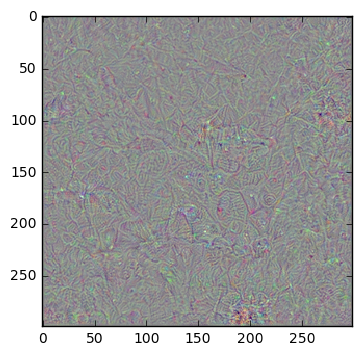## 练习

• 尝试为网络中较低层的特征运行多次优化。得到的图像总是相同吗？
• 试着用更少或更多的优化迭代。这对图像质量有何影响？
• 试着改变卷积特征的损失函数。这可以用不同的方法来做。它将如何影响图样模式？为什么？
• 你认为优化器除了增大我们想要最大化的那个特征之外，会放大其他特征吗？你要怎么度量这个？你确定优化器一次只会最大化一个特征吗？
• 试着同时最大化多个特征。
• 在MNIST数据集上训练一个小一点的网络，然后试着对特征和层次做可视化。会更容易在图像中看到图案吗？
• 试着实现上述论文中的方法。
• 试着用你自己的方法来改善优化的图像。
• 向朋友解释程序如何工作。